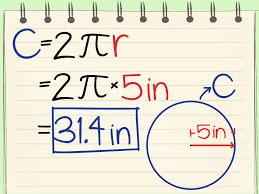# Welcome to my Website!

Welcome to the 3rd Javascript session today we will be learn how to find the value of PI and the circumference of a circle

The value of PI is

So most people know how to find the circumference of a circlethis is an image of the circumference of the circle the answer is 31.4in so we will now round that off

Now we have rounded off the answer so we got 31,in the image it shows the radius is 5in

so to end this session the value of PI is 3.14 and the answer to the circumference of a circle is 31.4in and the radius of the circle is 5in

This ends today's JavaScript session see all of you in the next Session if you missed the other session here is a link to my main website there you will find all of my session

go to main website